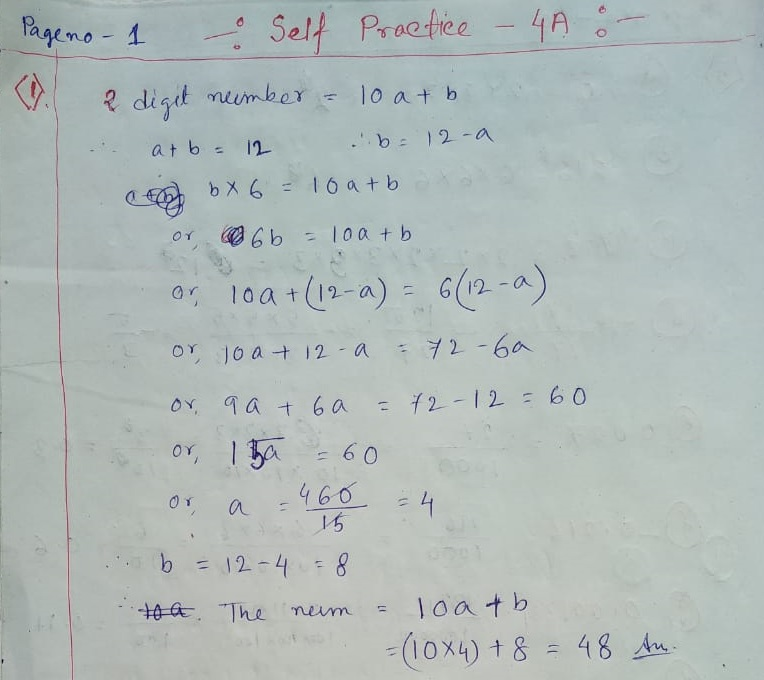# New Learning Composite Mathematics Class 8 SK Gupta Anubhuti Gangal Playing with Numbers Chapter 4A Solution

### New Learning Composite Mathematics SK Gupta Anubhuti Gangal Class 8 Playing with Numbers self Practice 4A Solution

(1) The sum of the digits of a 2-digit number is 12. The number is 6 times the units digit. Find the number.

SOLUTION Number 1:(2) The sum of the digits of a 2 digit number is 7. If the digits are reversed, the new number increased by 3 equals 4 times the original number. Find the original number.

(3) The sum of a number of two digits  and of the number formed by reversing the digits is 110 and the difference of the digits is 6. Find the number.

SOLUTION Number 2, 3:(4) A certain number between 10 and 100 is 8 times the sum of its digits. If 45 is subtracted from it the digits will be reversed. Find the number.

SOLUTION Number 4:(5) A number consists of three digits, the right hand being zero. if the left hand and the middle digits are interchanged the number is diminished by 180. If the left hand digit is halved and the middle and right hand digits are interchanged the number is diminished by 454. Find the number.

SOLUTION Number 5:Updated: January 23, 2020 — 11:33 am